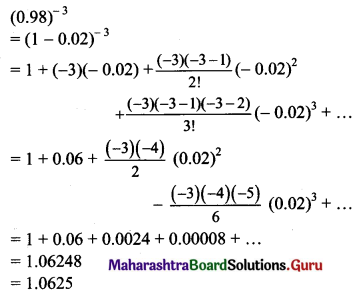# Maharashtra Board 11th Maths Solutions Chapter 4 Methods of Induction and Binomial Theorem Ex 4.4

Balbharti Maharashtra State Board 11th Maths Book Solutions Pdf Chapter 4 Methods of Induction and Binomial Theorem Ex 4.4 Questions and Answers.

## Maharashtra State Board 11th Maths Solutions Chapter 4 Methods of Induction and Binomial Theorem Ex 4.4

Question 1.
State, by writing the first four terms, the expansion of the following, where |x| < 1.
(i) (1 + x)-4
Solution: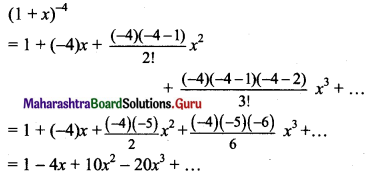(ii) (1 – x)1/3
Solution: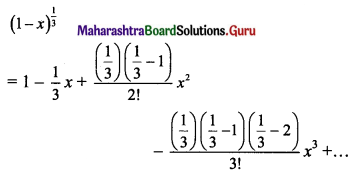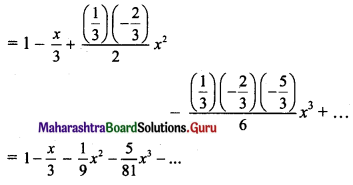(iii) (1 – x2)-3
Solution: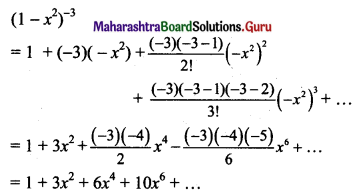(iv) (1 + x)-1/5
Solution: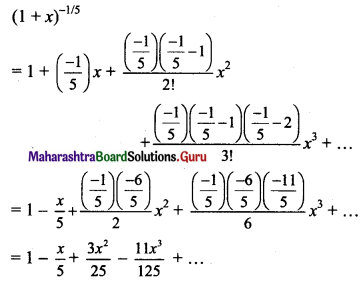(v) (1 + x2)-1
Solution: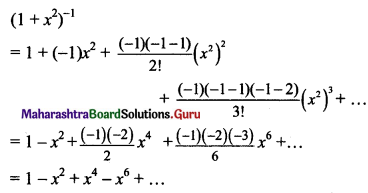Question 2.
State by writing first four terms, the expansion of the following, where |b| < |a|.
(i) (a – b)-3
Solution:
(a – b)-3 = $$\left[a\left(1-\frac{b}{a}\right)\right]^{-3}$$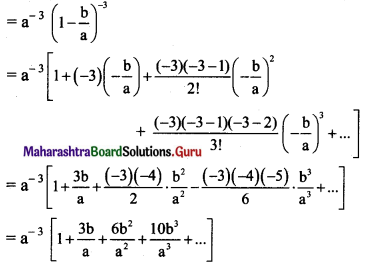(ii) (a + b)-4
Solution: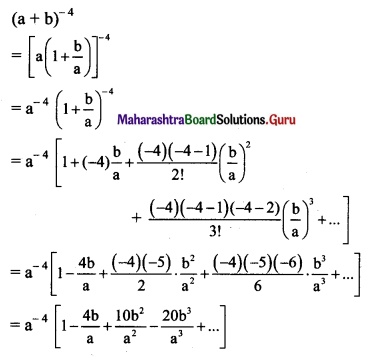(iii) (a + b)1/4
Solution: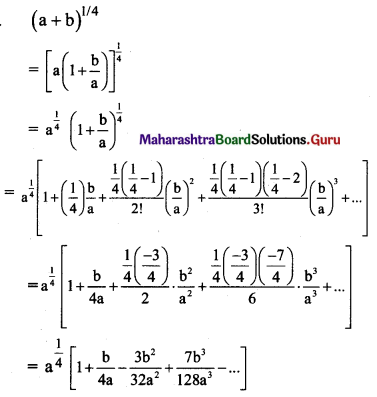(iv) (a – b)-1/4
Solution:
(a – b)-1/4 = $$\left[a\left(1-\frac{b}{a}\right)\right]^{\frac{-1}{4}}$$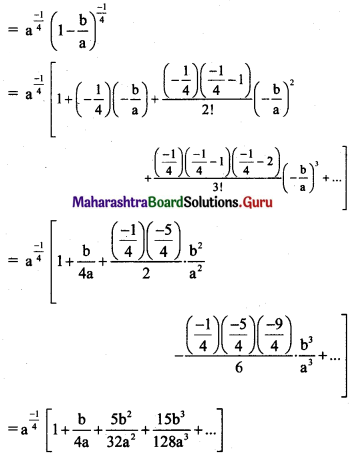(v) (a + b)-1/3
Solution: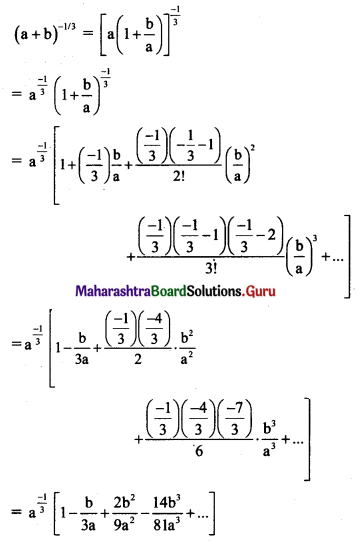Question 3.
Simplify the first three terms in the expansion of the following:
(i) (1 + 2x)-4
Solution: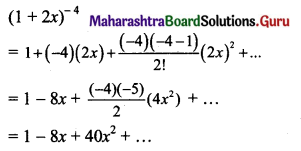(ii) (1 + 3x)-1/2
Solution: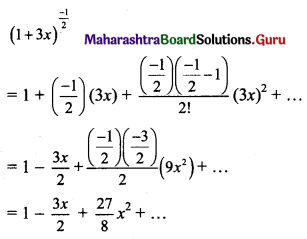(iii) (2 – 3x)1/3
Solution: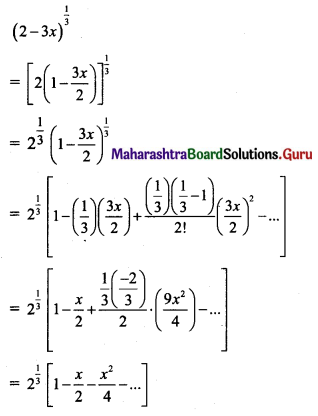(iv) (5 + 4x)-1/2
Solution: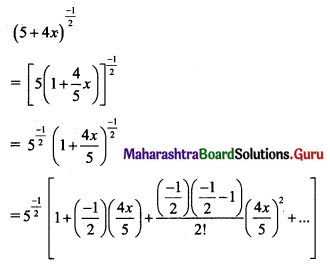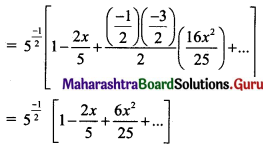(v) (5 – 3x)-1/3
Solution: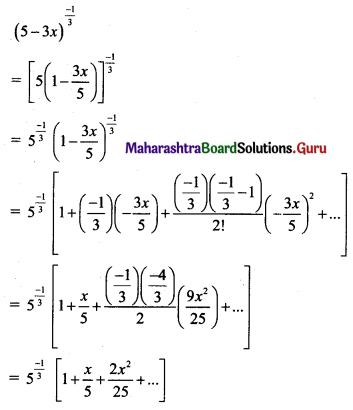Question 4.
Use the binomial theorem to evaluate the following upto four places of decimals.
(i) √99
Solution: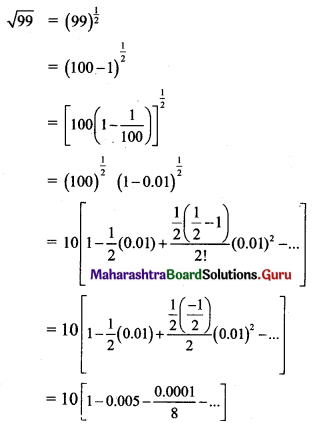= 10 [1 – 0.005 – 0.0000125 – ……]
= 10(0.9949875)
= 9.94987 5
= 9.9499

(ii) $$\sqrt{126}$$
Solution: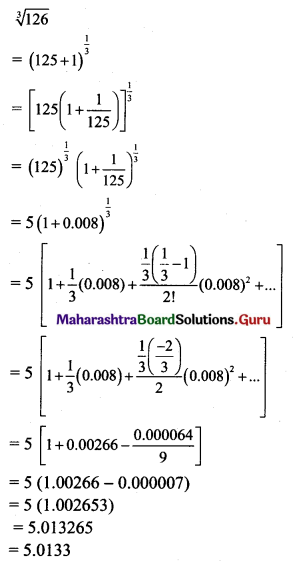(iii) $$\sqrt{16.08}$$
Solution: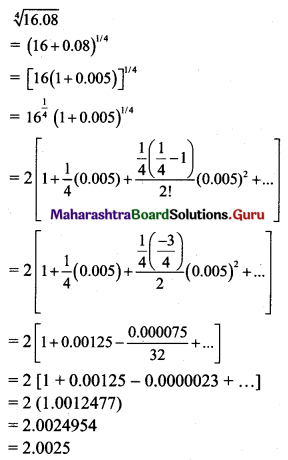(iv) (1.02)-5
Solution: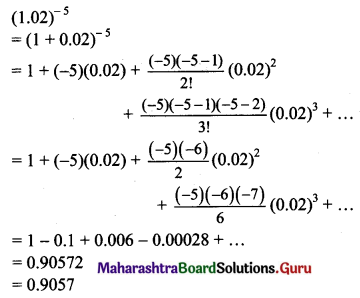(v) (0.98)-3
Solution: